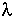## Technical Terms

Thermal conductivity () or Lambda value is expressend in W/mK.

It is the heat flow in Watts/m² for a temperature gradient of 1K per metre thickness.

The lower thevalue, the better the insulation.

Thermal resistance (R) expressed in m²K/W is the measure of resistance to the passage of heat for a given thickness t.

R = t/The higher the R value, the better the insulation

U value (or K value) expressed as W/m²K is the coefficient of thermal transmittance and is the reciprocal thermal resistance R so: U = 1/R

This is the amount of energy passing through a material or structure in one hour expressed in Watts per Metre² for a temperature difference between the two surfaces of 1C (=1 Kelvin).

Hence, the lower the U value, the better the insulation.

The U value of a system or structure is the reciprocal of the total thermal resistance value of each component element of the structure:
i.e. U = 1/R1 + R2 + R3 ... etc.

NOTE: since R = t () and U = 1/R

U = 1/t/( l ) which = 1 X ( l )

so

U = ()/t therefore when t = 1 metre, U =value.